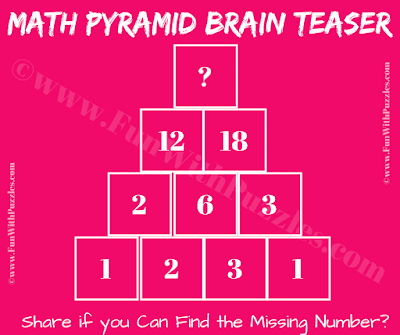This is an easy Pyramid Math Picture Puzzle Challenge to test your #logical #reasoning and mathematical skills. In this pyramid math puzzle, some numbers are arranged in a triangular shape. Use your logical reasoning skills to find the relationship between these numbers and then apply the same mathematical formula to find the value of the missing number which will replace the question mark at the top of the pyramid.Can you solve this Pyramid Math Picture Puzzle Challenge?

The answer to this "Pyramid Math Puzzle Challenge", can be viewed by clicking on the answer button.

Unknown said...

41